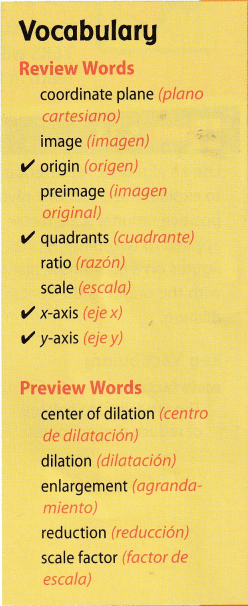# Texas Go Math Grade 8 Module 13 Answer Key Dilations, Similarity, and Proportionality

Refer to our Texas Go Math Grade 8 Answer Key Pdf to score good marks in the exams. Test yourself by practicing the problems from Texas Go Math Grade 8 Module 13 Answer Key Dilations, Similarity, and Proportionality.

## Texas Go Math Grade 8 Module 13 Answer Key Dilations, Similarity, and Proportionality

Write each ratio in the simplest form.

Question 1.
$$\frac{6}{15}$$ ____________
To write a ratio in simplest form, find the greatest factor of the numerator and denominator.
$$\frac{6}{15}=\frac{6 \div 3}{15 \div 3}$$ (Divide the numerator and denominator by the GCF)
= $$\frac{2}{5}$$

Question 2.
$$\frac{8}{20}$$ ____________
To write a ratio in simplest form, find the greatest factor of the numerator and denominator.
$$\frac{8}{20}=\frac{8 \div 4}{20 \div 4}$$ (Divide the numerator and denominator by the GCF)
= $$\frac{2}{5}$$

Question 3.
$$\frac{30}{18}$$ ____________
To write a ratio in simplest form, find the greatest factor of the numerator and denominator.
$$\frac{30}{18}=\frac{30 \div 6}{18 \div 6}$$ (Divide the numerator and denominator by the GCF)
= $$\frac{5}{3}$$

Question 4.
$$\frac{36}{30}$$ ____________
To write a ratio in simplest form, find the greatest factor of the numerator and denominator.
$$\frac{36}{30}=\frac{36 \div 6}{30 \div 6}$$ (Divide the numerator and denominator by the GCF)
= $$\frac{6}{5}$$

Find the perimeter.

Question 5.
square with sides of 8.9 cm
side = 8.9 cm
We know that,
Perimeter of the square = 4s
P = 4 × 8.9
P = 35.6 cm
Thus the perimeter of the square = 35.6 cm

Question 6.
rectangle with length 5$$\frac{1}{2}$$ ft and width 2$$\frac{3}{4}$$ ft
Given,
length 5$$\frac{1}{2}$$ ft and width 2$$\frac{3}{4}$$ ft
We know that,
Perimeter of the rectangle = 2L + 2W
P = 2 (5$$\frac{1}{2}$$ + 2$$\frac{3}{4}$$)
P = 2 ($$\frac{11}{2}$$ + $$\frac{11}{4}$$)
P = 2 ($$\frac{33}{4}$$
P = 33/2
P = 16 $$\frac{1}{2}$$
Thus the perimeter of the rectangle is 16 $$\frac{1}{2}$$ ft

Question 7.
equilateral triangle with sides of 8$$\frac{3}{8}$$ in.
Given,
s = 8$$\frac{3}{8}$$ in or 8.3
We know that,
Perimeter of the equilateral triangle = 3a
P = 3 × 8.3
P = 24.9 ft
Thus the perimeter of the equilateral triangle is 24.9 ft

Find the area.

Question 8.
Square with sides of 6.5 cm: ___________
Given,
side = 6.5 cm
We know that,
Area of a square = s × s
A = 6.5 × 6.5
A = 42.25 sq. cm
Thus the area of the square is 42.25 sq. cm

Question 9.
Triangle with base 10 in. and height 6 in.:
Given,
Triangle with base 10 in. and height 6 in
We know that,
Area of triangle = 1/2 × base × height
A = 1/2 × 10 × 6
A = 5 × 6
A = 30 sq. inches

Question 10.
Rectangle with length 3$$\frac{1}{2}$$ ft and width 2$$\frac{1}{2}$$ ft:
Given,
length 3$$\frac{1}{2}$$ ft and width 2$$\frac{1}{2}$$ ft
We know that,
Area of the rectangle = l × w
A = 3$$\frac{1}{2}$$ × 2$$\frac{1}{2}$$
A = $$\frac{7}{2}$$ × $$\frac{5}{2}$$
A = $$\frac{35}{4}$$
Thus the area of the rectangle is $$\frac{35}{4}$$ sq. ft

Visualize Vocabulary

Use the ✓ words to complete the graphic organizer. You will put one word in each rectangle.Understand Vocabulary

Complete the sentences using the review words.

Question 1.
A figure larger than the original, produced through dilation, is an ____________